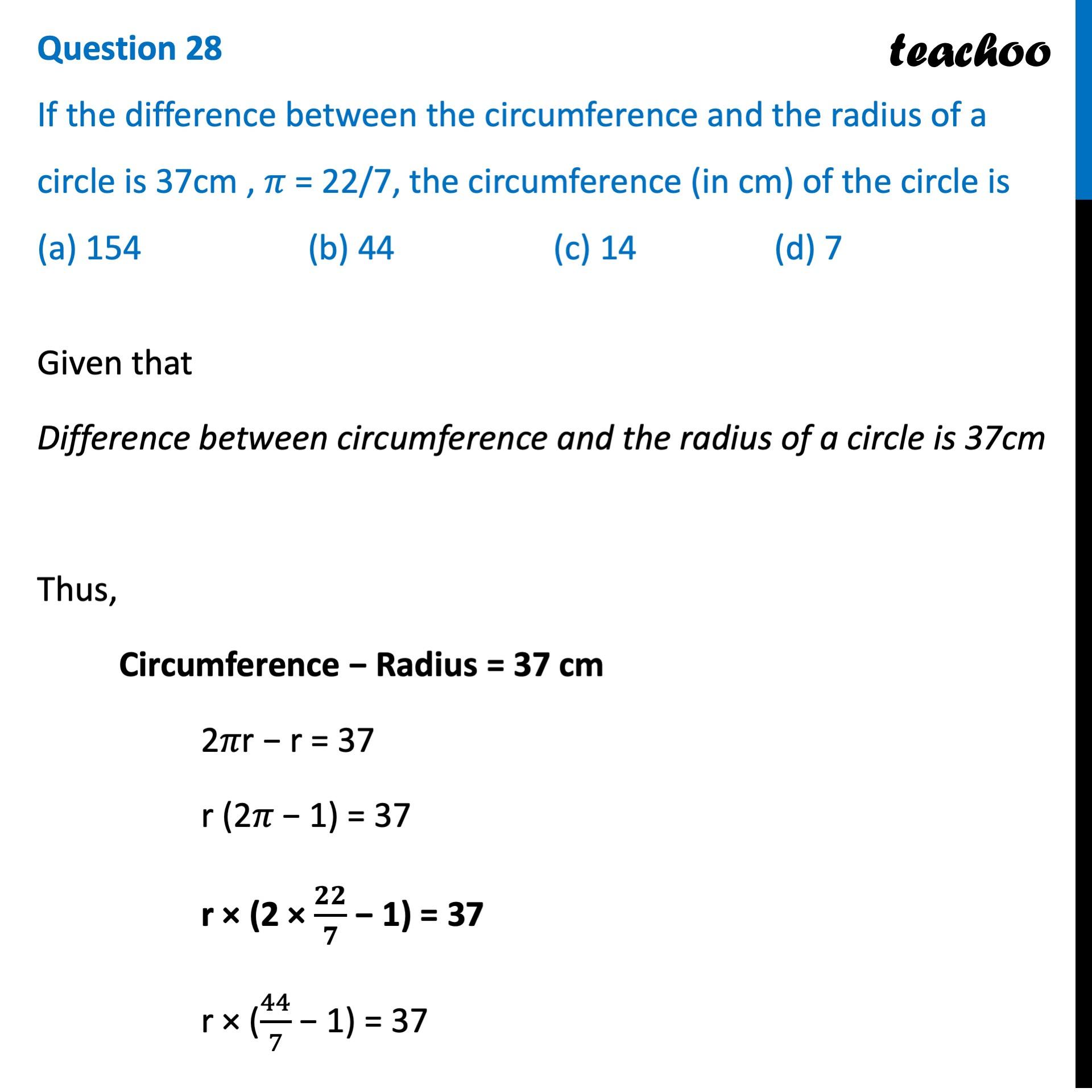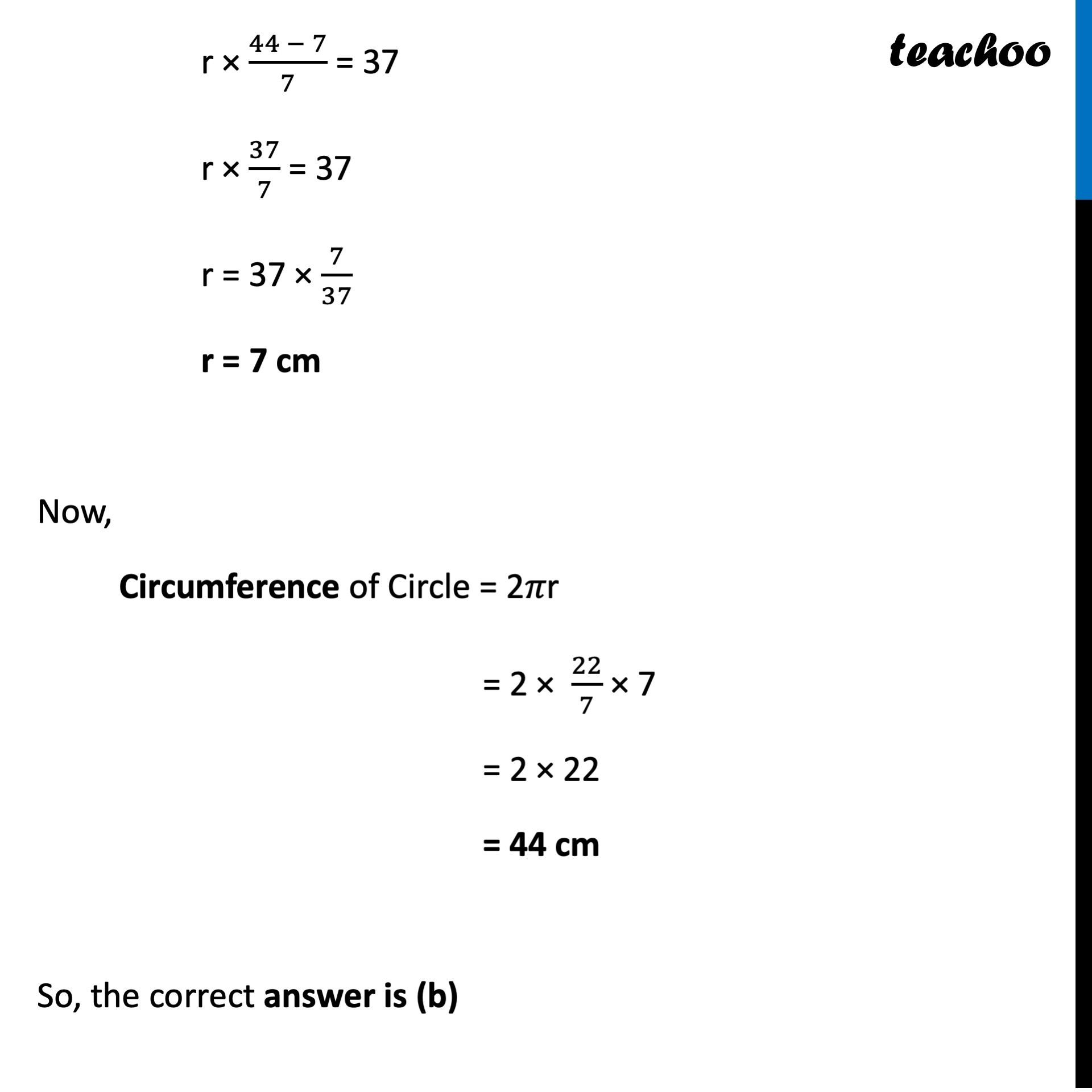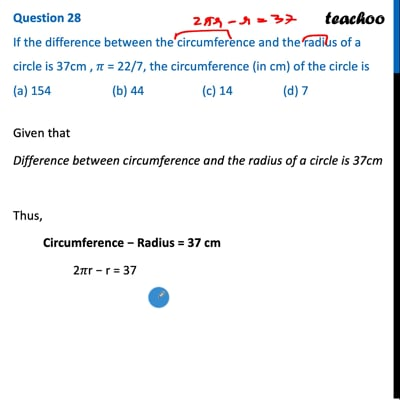CBSE Class 10 Sample Paper for 2022 Boards - Maths Basic [MCQ]

Class 10
Solutions of Sample Papers for Class 10 Boards

## (a) 154    (b) 44    (c) 14     (d) 7This video is only available for Teachoo black users

Introducing your new favourite teacher - Teachoo Black, at only ₹83 per month

### Transcript

Question 28 If the difference between the circumference and the radius of a circle is 37cm , 𝜋 = 22/7, the circumference (in cm) of the circle is Given that Difference between circumference and the radius of a circle is 37cm Thus, Circumference − Radius = 37 cm 2𝜋r − r = 37 r (2𝜋 − 1) = 37 r × (2 × 𝟐𝟐/𝟕 − 1) = 37 r × (44/7 − 1) = 37 r × (44 − 7)/7 = 37 r × 37/7 = 37 r = 37 × 7/37 r = 7 cm Now, Circumference of Circle = 2𝜋r = 2 × 22/7 × 7 = 2 × 22 = 44 cm So, the correct answer is (b)